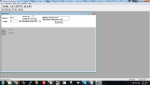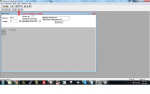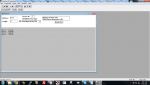# How to convert modbus 8byte hex to double

#### kannannatesh

Dear Experts,
Currently, i am trying to read Modbus data through Arduino i am getting FCF4D4823FF70001 Hex data from my sensor that value is 1.489383(double) but i can't understand how to convert the hex to double if anyone has knowledge in this conversion kindly let us know this will help me to complete the conversion

Note: this is my first project in Modbus integration with microcontroller

regards
kannan

•XiaoJun

#### jschulze

You need to confirm the format of the value from your sensor. A double is a 64-bit encoded value, which is very rare for Modbus devices. Are you sure you don't have a 32-bit floating point value?

Taking the least-significant 32-bits from your hex data, 3FF70001, and converting to decimal equates to a value of 1.92968761921, which is close to your expected value of 1.489383.

Can you share the Modbus documentation for the sensor you're trying to communicate with?

#### kannannatesh

You need to confirm the format of the value from your sensor. A double is a 64-bit encoded value, which is very rare for Modbus devices. Are you sure you don't have a 32-bit floating point value?

Taking the least-significant 32-bits from your hex data, 3FF70001, and converting to decimal equates to a value of 1.92968761921, which is close to your expected value of 1.489383.

Can you share the Modbus documentation for the sensor you're trying to communicate with?

Hi Jschulze
Thanks for your reply, We bought a flow sensor from a local distributor and they are imported from China so they are don't have much technical documents and they share single page register address with the data type, I have attached the sheet for your reference,
that sheet shows different register addresses and data types but if I am trying to read the value with Modbus software on PC that shows null except two registers 117, 109. As per the register map, 109 is +ve totalizer 117 is the net totalizer 117 hold 8 bytes double data that why I am asking the forum if you can help me to solve this conversion.

#### Attachments

• 343.2 KB Views: 15

#### XiaoJun

Dear Experts,
Currently, i am trying to read Modbus data through Arduino i am getting FCF4D4823FF70001 Hex data from my sensor that value is 1.489383(double) but i can't understand how to convert the hex to double if anyone has knowledge in this conversion kindly let us know this will help me to complete the conversion

Note: this is my first project in Modbus integration with microcontroller

regards
kannan
Modbus is a protocol of communication. It means you need to implement it in software, it is easy to implement or there are many libraries too in C. And RS485 is a differential serial communication standard which can be implemented by using 485 driver by interfacing it with MCU UART

#### kannannatesh

Modbus is a protocol of communication. It means you need to implement it in software, it is easy to implement or there are many libraries too in C. And RS485 is a differential serial communication standard which can be implemented by using 485 driver by interfacing it with MCU UART
Hi Xiaojun,
thanks for your reply, i have completed reading data from the Modbus meter with the help of MAX485, My problem is the meter returns 8byte double data, this is the return value in hex FCF4D4823FF70001 i don't know how to convert the hex data to double

#### jschulze

The Modbus mapping you attached shows that the flow meter's parameters are exposed three different ways, as doubles, floats, and signed long integers. For example, the Flow Rate can be accesses as a double by reading register addresses 105 - 108 (4 registers in total), as a float by reading register addresses 133 - 134 (2 registers in total), or as a signed long integer by reading register addresses 163 - 164 (2 registers in total).

I recommend that you try accessing the registers listed above to see if you can properly decode any of the three different encodings.

Additionally, please note that Modbus register addressing is not consistent among all vendors. Some vendors use a 0-based register address, while others use a 1-based register number. Therefore, you may need to add 1 to the register addresses that are listed in the flow meter's table when reading values using your Modbus software.

#### jschulze

After taking another look at the original values you listed when reading the double value, FCF4D4823FF70001 Hex and 1.489383 decimal, I noticed something that may help you decode the value.

A double value of 1.489383 in decimal corresponds with a hex representation of 3FF7 D482 FCF4 XXXX, after roudning, and where the XXXX just specifies additional lower significant digits. If you take your hex value and split it up into 16-bit register values, you get FCF4 D482 3FF7 0001.

Therefore, I believe you may be running into the "off-by-one" Modbus addressing issue and the 0001 portion of your value is from a different parameter. For example, the 16-bit register values you should see are XXXX FCF4 D482 3FF7, again where XXXX specifies additional lower significant digits. In addition, you need to reverse the register ordering of how you are interpreting the value so that the value becomes 3FF7 D482 FCF4 XXXX.

#### kannannatesh

Hi jschulze,
Thanks for the response, this is my complete data 010308FCF4D4823FF700013925 this data is received from my sensor, then i am a newbie to RS485 so can't understand the communication and other things, i was googled about data conversion i found this https://control.com/forums/threads/converting-hex-to-floating-point.33067/ this is for floating-point but i get double data that's why i asking to this forum,
my sensor gives output net totalizer value in only two registers 109-112 and 117-120 other registers are giving zero value that is the problem here and our supplier doesn't have a piece of technical knowledge about which we are bought from him, he is just a reseller/dealer. if you have time kindly explain the step by the procedure to convert the above hex and finally i want to show 1.489383 double value in my microcontroller.

#### jschulze

this is my complete data 010308FCF4D4823FF700013925 this data is received from my sensor
I understand that this is the sensor's raw Modbus response, but you don't state what registers you're reading to receive that data. Like I said before, I think you may need to add 1 to your register address (i.e. if you were using 109, you need to use 110 instead) and reverse the ordering of the registers (i.e. little endian vs. big endian).

All Modbus registers are 16-bits. In order to interpret the 32-bit or 64-bit values, multiple registers must be combined. Please tell me what registers you're reading and the 16-bit hex value of each register.

For example, starting at register 101, read 23 consecutive registers and list the values like this:

Register 101 = 12345 hex
Register 102 = 6789 hex
Register 103 = ABCD hex
Register 104 = EF01 hex
...
Register 122 = 2468 hex
Register 123 = ACE0 hex

Also, what is the Modbus software you're using?

And here is a converter you can use:
https://babbage.cs.qc.cuny.edu/IEEE-754/

#### kannannatesh

Hi jschulze
i have attached my modscan32 software screenshot for your reference and i am reading the Holding register 117, the screenshot shows i choose double variable i get the exact meter value which is shown in the meter display, otherwise, if I choose float type the software shows 1.9362 in 119 registers at same time 117register shows????? if I choose HEX variable that shows value what i am getting through my microcontroller#### jschulze

OK, so register 117 is encoded as a double data type and when you select the double data format in ModScan, you see the correct value. It seems that you got it working correctly.

Now, this is different than your original post, so what changed? Your original post had a hex value of FCF4D4823FF70001, but now you are showing a hex value of 1C49FCF4D4823FF7 (note that the register ordering is actually little endian - least significant register first, so the real hex value is 3FF7D482FCF41C49).

Were you using a different Modbus software that uses 0-based addressing (note that ModScan uses 1-based addressing)?

#### kannannatesh

Thanks for the clarification, i already mentioned i am a newbie to RS485 so if you can kindly help to how can i convert the hex data to double, i was searched in google regarding this finally i found this link
https://en.wikipedia.org/wiki/IEEE_754-1985#Single-precision_32-bit
it shows an explanation for 32 bit only but my data is 64bit what can i do for this situation

#### jschulze

If the Modbus library you're using on your Arduino uses 0-based addressing (which is how the address is encoded into the Modbus packet itself), make sure to start at register 116 instead of 117 so that you see the same hex value in your Arduino as ModScan showed.

You don't need to understand the specifics converting hex to double by hand. In your Arduino, you simply need to cast the data correctly. The trick is using a temporary 64-bit integer, casting it to a double pointer, and dereferencing it to get the value as a double. Here is example code:

C:
``````unsigned short reg_data;
long temp_int64;
double final_value;

// Read 4 holding registers from device address 1 starting at register 117 (0-base address of 116) and store in reg_data

// Copy the least significant 16-bit register
temp_int64 = (long)reg_data;

// Copy the next 16-bit register
temp_int64 = temp_int64 | (((long)reg_data) << 16);

// Copy the next 16-bit register
temp_int64 = temp_int64 | (((long)reg_data) << 32);

// Copy the most significant 16-bit register
temp_int64 = temp_int64 | (((long)reg_data) << 48);

// Convert the 64-bit integer to a double
final_value = *((double *)&temp_int64);``````

#### kannannatesh

If the Modbus library you're using on your Arduino uses 0-based addressing (which is how the address is encoded into the Modbus packet itself), make sure to start at register 116 instead of 117 so that you see the same hex value in your Arduino as ModScan showed.

You don't need to understand the specifics converting hex to double by hand. In your Arduino, you simply need to cast the data correctly. The trick is using a temporary 64-bit integer, casting it to a double pointer, and dereferencing it to get the value as a double. Here is example code:

C:
``````unsigned short reg_data;
long temp_int64;
double final_value;

// Read 4 holding registers from device address 1 starting at register 117 (0-base address of 116) and store in reg_data

// Copy the least significant 16-bit register
temp_int64 = (long)reg_data;

// Copy the next 16-bit register
temp_int64 = temp_int64 | (((long)reg_data) << 16);

// Copy the next 16-bit register
temp_int64 = temp_int64 | (((long)reg_data) << 32);

// Copy the most significant 16-bit register
temp_int64 = temp_int64 | (((long)reg_data) << 48);

// Convert the 64-bit integer to a double
final_value = *((double *)&temp_int64);``````
Thanks for the step by step clarification, then i don't use any library in my Arduino code i have attached my code for your reference, i will try above steps and i will let you know the result if you have knowledge on Arduino coding kindly help to understand one problem
i have used the serial write function for sending the command to RS485 then my meter also respond correctly, but if i use serial print function instead of serial write my meter doesn't respond, i know serial write send binary that's why i am using serial print(value,BIN); but that is not working

#include <SoftwareSerial.h>

#define Pin13LED 13
byte byteSend;
void setup()
{
Serial.begin(9600);
Serial1.begin(9600);
Serial.println("MODBUS...");
}
void loop()
{
while (Serial1.available())
{
Serial.print("DATA:");
Serial.println(byteSend, HEX);
}
delay(1000);
byte request[] = {0x01, 0x03, 0x00, 0x75, 0x00, 0x04, 0x55, 0xD3};
int i=0;
for(i=0; i<sizeof(request); i++)
{
// Serial1.write(request);
Serial1.print(request,BIN);
}

#### jschulze

I'm sorry, I'm not very familiar with the Arduino's libraries, but a Google search turns up this as the first result:
https://arduino.stackexchange.com/q...rial-write-and-serial-print-and-when-are-they

According to that link, you need to use Serial.write to send packets to your sensor, since Modbus RTU uses binary encoding. You would only use Serial.print when printing ASCII characters to a console or if you were doing Modbus ASCII instead of Modbus RTU.

Additionally, as I stated in my last reply, you need to use register address 116 not 117 in the packet. Therefore, you need to change the 0x75 to 0x74 in your request[] initialization.

#### kannannatesh

Sure, i will change the register value 116 instead of 117, then i will update the result to the forum after converting data, i am just using Arduino for check my meter can talk with my microcontroller or not, if i am complete the conversion with my Arduino, i will change the controller that's why i ask how to use a serial print function instead of serial write

#### jschulze

There is one additional thing to watch out for, since you are reading the packet manually, byte-by-byte and not using a Modbus library.

Each 16-bit Modbus register uses big-endian byte ordering, i.e. high byte then low byte, as per the Modbus specification. But the ordering of the 16-bit registers are little-endian word ordering when combining the 16-bit registers to get the 64-bit value.

So you'll need to do something like this to get each 16-bit register value:
C:
``reg_data = (((unsigned short)data_byte) << 8) | ((unsigned short)data_byte);``
Then you can combined the 16-bit registers as I showed in my previous example.

#### kannannatesh

There is one additional thing to watch out for, since you are reading the packet manually, byte-by-byte and not using a Modbus library.

Each 16-bit Modbus register uses big-endian byte ordering, i.e. high byte then low byte, as per the Modbus specification. But the ordering of the 16-bit registers are little-endian word ordering when combining the 16-bit registers to get the 64-bit value.

So you'll need to do something like this to get each 16-bit register value:
C:
``reg_data = (((unsigned short)data_byte) << 8) | ((unsigned short)data_byte);``
Then you can combined the 16-bit registers as I showed in my previous example.
i have shared the code what i am going to upload to arduino for your reference, if i miss any step what you are share with me kindly point that mistake

#include <SoftwareSerial.h>

#define Pin13LED 13
byte byteSend;

unsigned short reg_data;
long temp_int64;
double final_value;
int j=0;

void setup()
{
Serial.begin(9600);
Serial1.begin(9600);
Serial.println("MODBUS Test...");
}
void loop()
{
while (Serial1.available())
{
j++;
Serial.print("DATA:");
Serial.println(byteSend[j], HEX);
}
reg_data = (((unsigned short)byteSend) << 8) | ((unsigned short)byteSend);
reg_data = (((unsigned short)byteSend) << 8) | ((unsigned short)byteSend);
reg_data = (((unsigned short)byteSend) << 8) | ((unsigned short)byteSend);
reg_data = (((unsigned short)byteSend) << 8) | ((unsigned short)byteSend);

// Copy the least significant 16-bit register
temp_int64 = (long)reg_data;

// Copy the next 16-bit register
temp_int64 = temp_int64 | (((long)reg_data) << 16);

// Copy the next 16-bit register
temp_int64 = temp_int64 | (((long)reg_data) << 32);

// Copy the most significant 16-bit register
temp_int64 = temp_int64 | (((long)reg_data) << 48);

// Convert the 64-bit integer to a double
final_value = *((double *)&temp_int64);

Serial.print("Double Data:");
Serial.println(final_value);

delay(1000);
j=0;
byte request[] = {0x01, 0x03, 0x00, 0x74, 0x00, 0x04, 0x55, 0xD3};
int i=0;
for(i=0; i<sizeof(request); i++)
{
Serial1.write(request);
//Serial1.print(request,BIN);
}
}

#### jschulze

You're not copying the correct data bytes from the received packet.

You need to change these lines:
C:
``````reg_data = (((unsigned short)byteSend) << 8) | ((unsigned short)byteSend);
reg_data = (((unsigned short)byteSend) << 8) | ((unsigned short)byteSend);
reg_data = (((unsigned short)byteSend) << 8) | ((unsigned short)byteSend);
reg_data = (((unsigned short)byteSend) << 8) | ((unsigned short)byteSend);``````
To this:
C:
``````reg_data = (((unsigned short)byteSend) << 8) | ((unsigned short)byteSend);
reg_data = (((unsigned short)byteSend) << 8) | ((unsigned short)byteSend);
reg_data = (((unsigned short)byteSend) << 8) | ((unsigned short)byteSend);
reg_data = (((unsigned short)byteSend) << 8) | ((unsigned short)byteSend);``````

Also, you're printing the read byte after incrementing j. These lines:
C:
``````while (Serial1.available())
{
j++;
Serial.print("DATA:");
Serial.println(byteSend[j], HEX);
}``````
Should be this:
C:
``````while (Serial1.available())
{
Serial.print("DATA:");
Serial.println(byteSend[j], HEX);
j++;
}``````

And as a general suggestion, since the Arduino is the master, it should send its request first, then read the response from the sensor. You have this backwards.

#### kannannatesh

You're not copying the correct data bytes from the received packet.

You need to change these lines:
C:
``````reg_data = (((unsigned short)byteSend) << 8) | ((unsigned short)byteSend);
reg_data = (((unsigned short)byteSend) << 8) | ((unsigned short)byteSend);
reg_data = (((unsigned short)byteSend) << 8) | ((unsigned short)byteSend);
reg_data = (((unsigned short)byteSend) << 8) | ((unsigned short)byteSend);``````
To this:
C:
``````reg_data = (((unsigned short)byteSend) << 8) | ((unsigned short)byteSend);
reg_data = (((unsigned short)byteSend) << 8) | ((unsigned short)byteSend);
reg_data = (((unsigned short)byteSend) << 8) | ((unsigned short)byteSend);
reg_data = (((unsigned short)byteSend) << 8) | ((unsigned short)byteSend);``````

Also, you're printing the read byte after incrementing j. These lines:
C:
``````while (Serial1.available())
{
j++;
Serial.print("DATA:");
Serial.println(byteSend[j], HEX);
}``````
Should be this:
C:
``````while (Serial1.available())
{